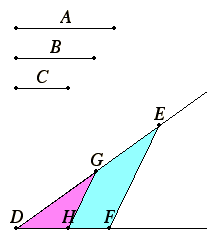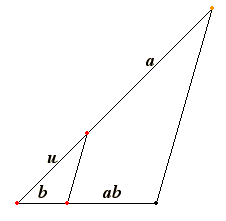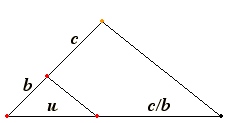# Proposition 12

To find a fourth proportional to three given straight lines.

Let A and B and C be the three given straight lines.It is required to find a fourth proportional to A, B, and C.

Set out two straight lines DE and DF containing any angle EDF. Make DG equal to A, GE equal to B, and DH equal to C. Join GH, and draw EF through E parallel to it.

VI.2

Then since GH is parallel to a side EF of the triangle DEF, therefore DG is to GE as DH is to HF.

V.7

But DG equals A and GE to B, and DH to C, therefore A is to B as C is to HF.

Therefore a fourth proportional HF has been found to the three given straight lines A, B, and C.

Q.E.F.

## Guide

Of course, the previous proposition is a special case of this one.

#### Descartes’ geometric algebra

Descartes (1591–1661) is well known for his coordinate geometry which he and Fermat developed in the 16th century. This subject, also called analytic geometry, places an x-y-coordinate system on a plane so that a curve in the plane corresponds to an equation in two variables x and y. The usual way this correspondence is used is to convert a problem in geometry into an algebraic problem about equations.

Descartes was equally interested is using geometry to solve algebraic problems, but using a method quite distinct from that in the Elements which began in Book II.

His idea was to take an equation in one variable and find a geometric figure which can be used to solve the equation. The idea wasn’t particularly new as even Menaechmus (fl. about 350 B.C.E) had about 50 years before Euclid intersected two parabolas to find cube roots, which are solutions to particular cubic equations. Furthermore, about 1100, Omar Khayyam solved all cubic equations by means of parabolas and hyperbolas. But Descartes was systematic and was able to use the relatively recent invention of symbolic algebra to make more connections.

Descartes began by interpreting the algebraic operations of addition, subtraction, multiplication, division, and extraction of square roots as geometric constructions on lines. He represented each (positive) magnitude by a line. Addition and subtraction were the same as Euclid’s. To add two lines, just extend one by the length of the other. To subtract one line from another, just take the remainder after cutting it off the other. Multiplication and division, however, were different from Euclid’s. Euclid represented the product of two lines by a rectangle, the product of three lines by a box in space, and Euclid didn’t represent the product of four lines.
 But Descartes took the product of two lines to be another line. That required selecting a unit line, that is, a line of length 1. Then to find the product ab of two quantities a and b, he only needed to find the fourth proportional of 1, a, and b. This proposition VI.10 does that. In the diagram to the right, u is the unit line, a and b are to be multiplied, and ab is their product, the fourth proportional.This same proposition works to construct the quotient of two quantities. If b and c are two quantities, then the fourth proportional for b, c, and 1 is the quotient c/b. Descartes achieved the fifth operation, extraction of square roots, by means of the semicircle and right angle construction described in the next proposition VI.13.#### Use of this proposition

This proposition is used in the proofs of VI.22, VI.23, and half a dozen propositions in Book X.